Research papers and code for "Kyle Helfrich":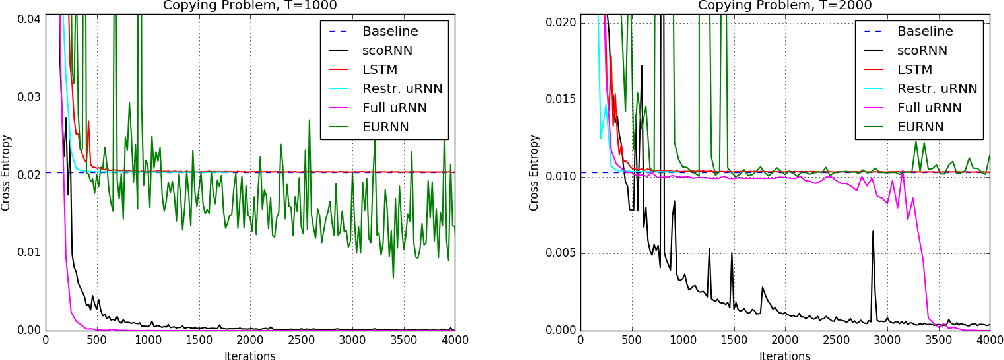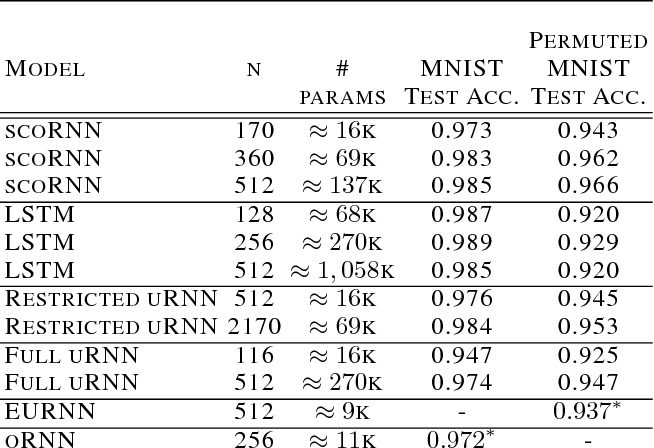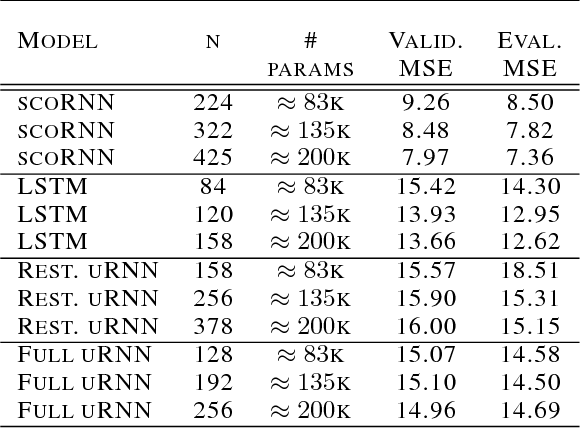Recurrent Neural Networks (RNNs) are designed to handle sequential data but suffer from vanishing or exploding gradients. Recent work on Unitary Recurrent Neural Networks (uRNNs) have been used to address this issue and in some cases, exceed the capabilities of Long Short-Term Memory networks (LSTMs). We propose a simpler and novel update scheme to maintain orthogonal recurrent weight matrices without using complex valued matrices. This is done by parametrizing with a skew-symmetric matrix using the Cayley transform. Such a parametrization is unable to represent matrices with negative one eigenvalues, but this limitation is overcome by scaling the recurrent weight matrix by a diagonal matrix consisting of ones and negative ones. The proposed training scheme involves a straightforward gradient calculation and update step. In several experiments, the proposed scaled Cayley orthogonal recurrent neural network (scoRNN) achieves superior results with fewer trainable parameters than other unitary RNNs.

* 12 pages
Click to Read Paper and Get Code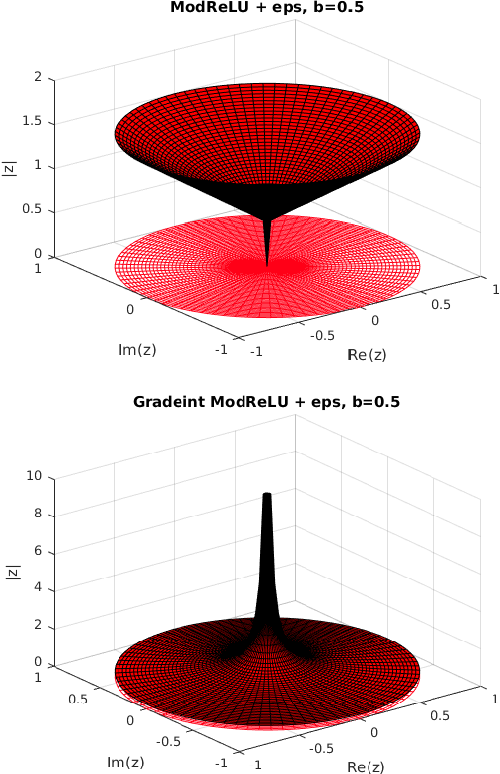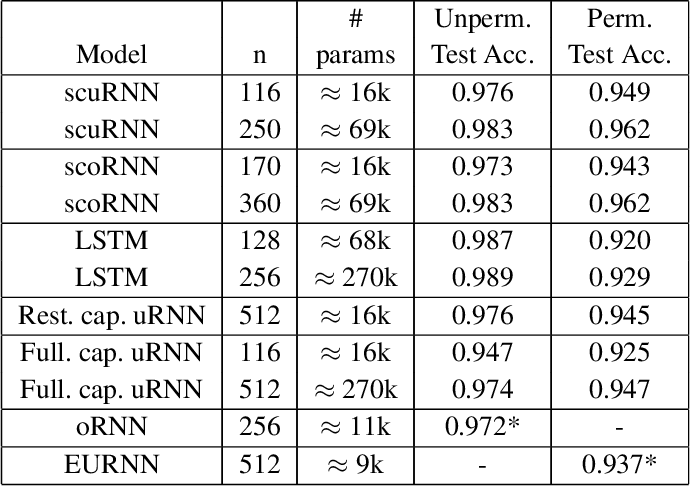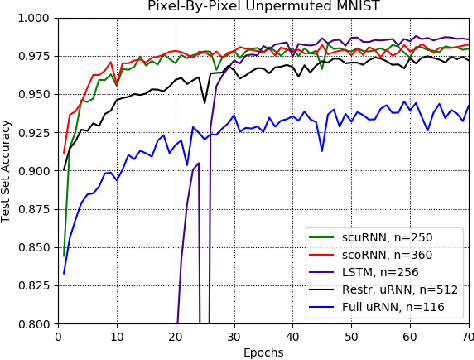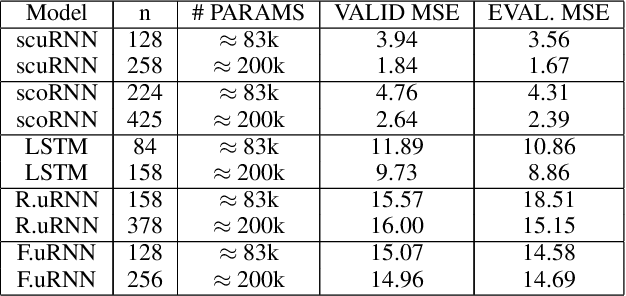Recurrent neural networks (RNNs) have been successfully used on a wide range of sequential data problems. A well known difficulty in using RNNs is the \textit{vanishing or exploding gradient} problem. Recently, there have been several different RNN architectures that try to mitigate this issue by maintaining an orthogonal or unitary recurrent weight matrix. One such architecture is the scaled Cayley orthogonal recurrent neural network (scoRNN) which parameterizes the orthogonal recurrent weight matrix through a scaled Cayley transform. This parametrization contains a diagonal scaling matrix consisting of positive or negative one entries that can not be optimized by gradient descent. Thus the scaling matrix is fixed before training and a hyperparameter is introduced to tune the matrix for each particular task. In this paper, we develop a unitary RNN architecture based on a complex scaled Cayley transform. Unlike the real orthogonal case, the transformation uses a diagonal scaling matrix consisting of entries on the complex unit circle which can be optimized using gradient descent and no longer requires the tuning of a hyperparameter. We also provide an analysis of a potential issue of the modReLU activiation function which is used in our work and several other unitary RNNs. In the experiments conducted, the scaled Cayley unitary recurrent neural network (scuRNN) achieves comparable or better results than scoRNN and other unitary RNNs without fixing the scaling matrix.

Click to Read Paper and Get Code